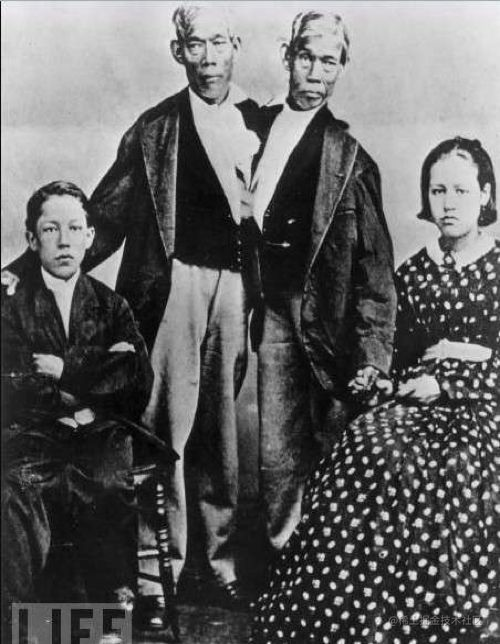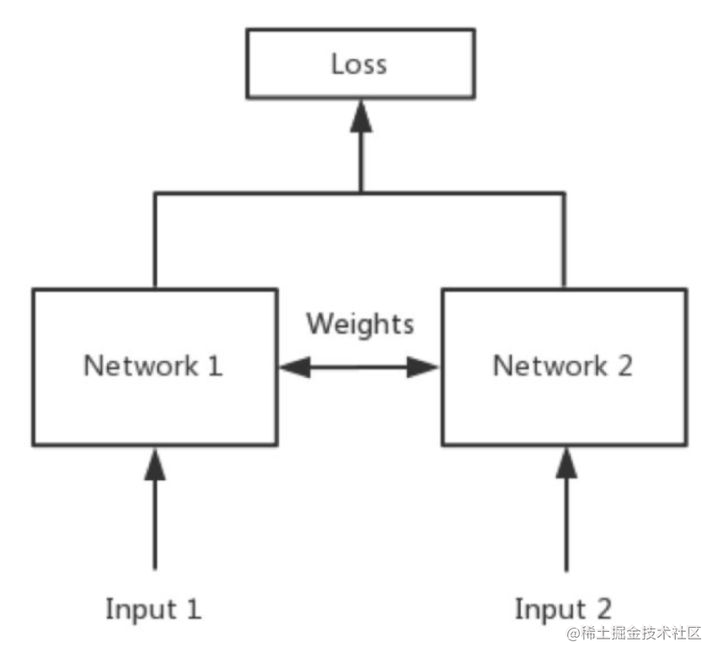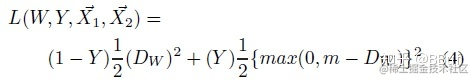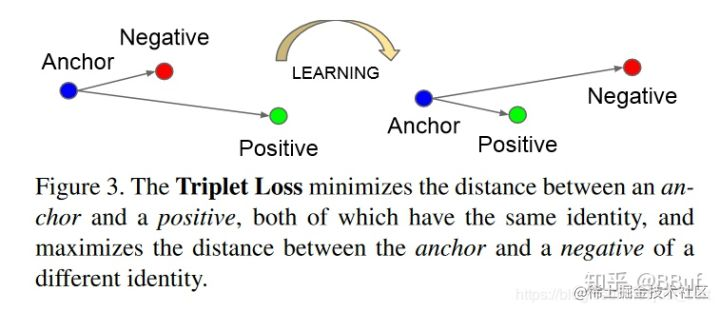# 孪生网络入门（上） Siamese Net

## 1 名字的由来## 2 模型结构• 其中的Network1和Network2按照专业的话来说就是共享权制，说白了这两个网络其实就是一个网络，在代码中就构建一个网络就行了；
• 一般的任务，每一个样本经过模型得到一个模型的pred，然后这个pred和ground truth进行损失函数的计算，然后得到梯度；这个孪生网络则改变了这种结构，假设是图片分类的任务，把图片A输入到模型中得到了一个输出pred1，然后我再把图片B输入到模型中，得到了另外一个输出pred2，然后我这个损失函数是从pred1和pred2之间计算出来的。 就是一般情况下，模型运行一次，给出一个loss，但是在siamese net中，模型要运行两次才能得到一个loss。
• 我个人感觉，一般的任务像是衡量一种绝对的距离，样本到标签的一个距离；但是孪生网络衡量的是样本到样本之间的一个距离。

### 2.1 孪生网络的用途

Siamese net衡量的是两个输入的关系，也就是两个样本相似还是不相似。

## 3 损失函数

• Contrastive Loss
• Triplte Loss

### 3.1 Contrastive Loss

• 提出论文：《Dimensionality Reduction by Learning an Invariant Mapping》

• 图片1 经过模型 得到pred1
• 图片2 经过模型 得到pred2
• pred1和pred2计算得到loss

• Y=0的时候，损失为：$（1-Y）L_S(D_W^i)$
• Y=1的时候，损失为：$YL_D(D_W^i)$.
• 其中论文中$L_D,L_S$是常数，论文中默认取0.5
• i是一个次方的含义，论文中和常用的contrastive loss中，都是默认i=2，也就是欧氏距离的平方。
• 对于类别是1（different类别的），我们自然是希望pred1和pred2的欧氏距离越大越好。那么这个大到什么程度是个头呢？损失函数是往小的方向移动，那么需要做什么呢？增加一个margin，当作最大的距离。如果pred1和pred2的距离大于margin，那么就认为这两个样本距离足够大，就当其的损失为0。所以写的方法就是：$max(margin-distance,0)$.
• 上图中的W我理解为神经网络的weight，然后$\vec X_1$，表示要输入的原图片。### 3.2 Contrastive Loss pytorch

# Custom Contrastive Loss
class ContrastiveLoss(torch.nn.Module):
"""
Contrastive loss function.
"""

def __init__(self, margin=2.0):
super(ContrastiveLoss, self).__init__()
self.margin = margin

def forward(self, output1, output2, label):
euclidean_distance = F.pairwise_distance(output1, output2)
loss_contrastive = torch.mean((1-label) * torch.pow(euclidean_distance, 2) +     # calmp夹断用法
(label) * torch.pow(torch.clamp(self.margin - euclidean_distance, min=0.0), 2))

return loss_contrastive

import torch
import torch.nn.functional as F
a = torch.Tensor([[1,2],[3,4]])
b = torch.Tensor([[10,20],[30,40]])
F.pairwise_distance(a,b)

### 3.3 Triplte Loss

• 提出论文：《FaceNet: A Unified Embedding for Face Recognition and Clustering》

• Triplet Loss定义：最小化锚点和具有相同身份的正样本之间的距离，最小化锚点和具有不同身份的负样本之间的距离。这个其实应该是三胞胎网络的损失函数，同时输入三个样本，一个图片，然后一个same类别的图片和一个different图片。
• Triplet Loss的目标：Triplet Loss的目标是使得相同标签的特征在空间位置上尽量靠近，同时不同标签的特征在空间位置上尽量远离，同时为了不让样本的特征聚合到一个非常小的空间中要求对于同一类的两个正例和一个负例，负例应该比正例的距离至少远margin。如下图所示：• 让anchor和positive得到的向量的欧氏距离越小越好；
• 让anchor和negative得到的向量的欧氏距离越大越好；

• 简单的说就是anchor和positive的距离要比anchor和negative的距离小，而且这个差距要至少要大于$\alpha$个人的思考是，这里的T，是三元组的集合。对于一个数据集，往往可以构建出非常多的三元组，因此我个人感觉这种任务一般用在类别多，数据量较少的任务中，不然三元组数量爆炸了

### 3.4 Triplte Loss keras

def triplet_loss(y_true, y_pred):
"""
Triplet Loss的损失函数
"""

anc, pos, neg = y_pred[:, 0:128], y_pred[:, 128:256], y_pred[:, 256:]

# 欧式距离
pos_dist = K.sum(K.square(anc - pos), axis=-1, keepdims=True)
neg_dist = K.sum(K.square(anc - neg), axis=-1, keepdims=True)
basic_loss = pos_dist - neg_dist + TripletModel.MARGIN

loss = K.maximum(basic_loss, 0.0)

print "[INFO] model - triplet_loss shape: %s" % str(loss.shape)
return loss

 Momentum Contrast for Unsupervised Visual Representation Learning, 2019, Kaiming He Haoqi Fan Yuxin Wu Saining Xie Ross Girshick

 Dimensionality Reduction by Learning an Invariant Mapping, 2006, Raia Hadsell, Sumit Chopra, Yann LeCun